• 多参数优化算法有哪些
千次阅读
2021-04-26 13:59:20

% 计算函数y的雅克比矩阵，是解析式

syms k a b R E T PH PCO y; % 定义函数和变量，共9个

y=k*exp(-E/(R*T))*PH^a*PCO^b; % 输入模型函数

R=8.314;

Jsym=jacobian(y,[k a b]); % 函数y的雅克比矩阵

% 拟合用数据。

% 2. LM算法

% 初始猜测s

k0=1.23*10^7; E0=93.18; a0=0.60; b0=-0.14;  % 设定初始猜测值

y_init = k0*exp(-E0/(R*data_3))*(data_1.^a0)*(data_2.^b0);  % 根据初始值及函数初步求解,注意变量指数形式为(.^)

% 数据个数

Ndata=length(obs_1);

% 参数维数

Nparams=3;

% 迭代最大次数

n_iters=50;

% LM算法的阻尼系数初值

lamda=0.01;

% step1: 变量赋值

updateJ=1;

k_est=k0;

a_est=a0;

b_est=b0;

% step2: 迭代

for it=1:n_iters

if updateJ==1

% 根据当前估计值，计算雅克比矩阵

J=zeros(Ndata,Nparams);

for i=1:length(data_1)

J(i,:)=[(data_2(i).^b_est)*(data_1(i).^a_est)*exp(-E/(R*data_3(i))) (data_2(i).^b_est)*(data_1(i).^a_est)*k_est*exp(-E/(R*data_3(i)))*log(data_1(i)) (data_2(i).^b_est)*(data_1(i).^a_est)*k_est*exp(-E/(R*data_3(i)))*log(data_2(i))]; %对k,a,b分别求导，原函数y=k*exp(-E/(R*T))*PH^a*PCO^b,即第

end

% 根据当前参数，得到函数值

y_est=k_est*exp(-E/(R*data_3(i)))*(data_1(i).^a_est)* (data_2(i).^b_est);

% 计算误差

d=obs_1-y_est;

% 计算(拟)海塞矩阵

H=J'*J;

% 若是第一次迭代，计算误差

if it==1

e=dot(d,d);

end

end

% 根据阻尼系数lamda混合得到H矩阵

H_lm=H+(lamda*eye(Nparams,Nparams));

% 计算步长dp，并根据步长计算新的可能的\参数估计值

dp=inv(H_lm)*(J'*d(:));

g = J'*d(:);

k_lm=a_est+dp(1); %变量k新的估计值

a_lm=a_est+dp(2); %变量a新的估计值

b_lm=b_est+dp(3); %变量b新的估计值

% 计算新的可能估计值对应的y和计算残差e

y_est_lm =k_lm*exp(-E/(R*data_3(i)))*(data_1(i).^a_lm)* (data_2(i).^b_lm);

d_lm=obs_1-y_est_lm;

e_lm=dot(d_lm,d_lm);

% 根据误差，决定如何更新参数和阻尼系数

if e_lm

lamda=lamda/10;

a_est=a_lm;

b_est=b_lm;

e=e_lm;

disp(e);

updateJ=1;

else

updateJ=0;

lamda=lamda*10;

end

end

%显示优化的结果

k_est

a_est

b_est

更多相关内容
• 最近发现果蝇算法优化参数效果，整理了果蝇算法优化一个以及参数的例程，在网上也搜索过，不过感觉大部分介绍的比较散乱，因此整理成为一个压缩包，希望对使用果蝇算法的人帮助！
• 基于遗传算法优化多尺度排列熵参数，类似于粒子群算法优化参数
• 目标遗传算法优化PID参数,是M文件。内容很详细，需要的可以下载
• 针对切削参数优化问题,以生产成本最小化为优化目标,基于斐波那契最优化原理与黄金分割,提出斐波那契树优化算法(FTO)进行优化求解.该算法通过全局探索与局部寻优交替进行,达到快速收敛到全局最优解的目的,避免...
• 建立了以最大生产效率和最低加工成本为目标优化的数学模型,采用遗传算法与Matlab工具实现对切削参数进行优化,得到目标函数的最优切削参数,并用实验验证提高数控机床加工效率和降低加工成本的可行性。
• 该代码通过引入鲸鱼优化算法（Whale Optimization Algorithm，WOA），实现对 libsvm 工具箱中的SVDD算法的参数优化，给出两个实例代码： 1. libsvm 工具箱提供的heart_scale data 2. 工业过程数据 WOA的具体描述...
• 梯度，共轭梯度，牛顿，拟牛顿，蒙特卡洛、Steepest Descent（SD）、L-BFGS等参数优化方法。 参数优化目标 在机器学习中，在定义好损失函数好，希望通过参数优化，使得损失函数最小。 2 梯度下降（最...

### 1. 分类

梯度法，共轭梯度法，牛顿法，拟牛顿法，蒙特卡洛法、Steepest Descent（SD）、L-BFGS等参数优化方法。

参数优化目标
在机器学习中，在定义好损失函数好，希望通过参数优化，使得损失函数最小。

### 2. 梯度下降法（最速下降法）

沿着梯度向量的方向，更加容易找到函数的最大值。反过来说，沿着梯度向量相反的方向（去负号），则就是更加容易找到函数的最小值。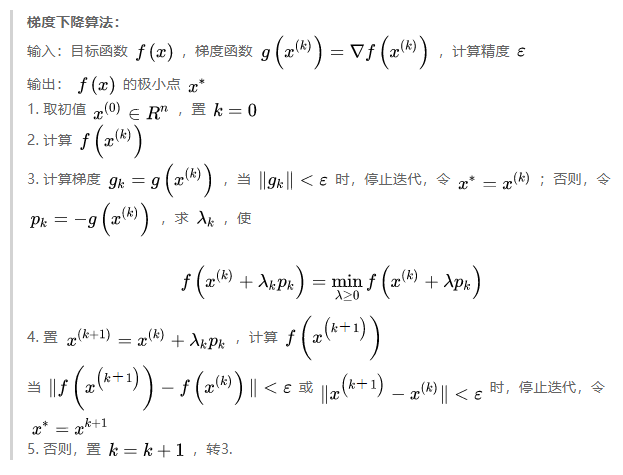里边的 λ k \lambda_k 是步长，常见是指定一个固定的比较小的常数，比如0.0001.

梯度下降法还可以分成：

在更新参数时使用所有的样本来进行更新。
θ = θ − η ⋅ ∇ θ J ( θ ) \theta = \theta - \eta \cdot \nabla_\theta J( \theta)

这里的 θ \theta 可以看做是上述梯度下降法中的 x x .

θ = θ − η ⋅ ∇ θ J ( θ ; x ( i ) ; y ( i ) ) \theta = \theta - \eta \cdot \nabla_\theta J( \theta; x^{(i)}; y^{(i)})
随机梯度下降法，与求梯度时没有用所有的样本的数据，而是仅仅选取一个样本来求梯度。

随机梯度下降法由于每次仅仅采用一个样本来迭代，训练速度很快，但仅仅用一个样本决定梯度方向，导致解很有可能不是最优，由于随机梯度下降法一次迭代一个样本，导致迭代方向变化很大，不能很快的收敛到局部最优解。

θ = θ − η ⋅ ∇ θ J ( θ ; x ( i : i + n ) ; y ( i : i + n ) ) \theta = \theta - \eta \cdot \nabla_\theta J( \theta; x^{(i:i+n)}; y^{(i:i+n)})

小批量梯度下降法是批量梯度下降法和随机梯度下降法的折衷，用一部分样本进行梯度计算。

缺点

• 靠近极小值时速度减慢。
• 直线搜索可能会产生一些问题。
• 可能会“之字型”地下降。

拓展：steepest descent
steepest descent,最陡下降。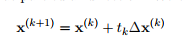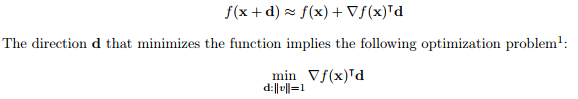d 选取 2范数则为梯度下降：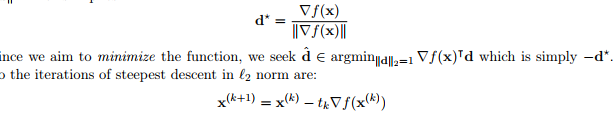也可以选取 1 范数（ coordinate descent）：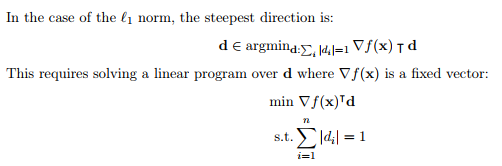### 3. 牛顿法

牛顿法的基本思想是利用目标函数的二次Taylor展开，并将其极小化。牛顿法主要应用在两个方面，1：求方程的根；2：最优化。

(1) 求方程的根
牛顿法利用一阶泰勒展开：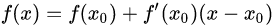迭代方式：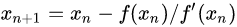迭代后求得方程的根：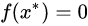为什么这里迭代公式能够保证二次收敛到方程的根，可以看证明：Quadratic Convergence of Newton’s Method Michael Overton, Numerical Computing, Spring 2017 .

(2) 最优化（求取极值）
无约束最优化问题：x ∗ x^{*} 为目标函数的极小点。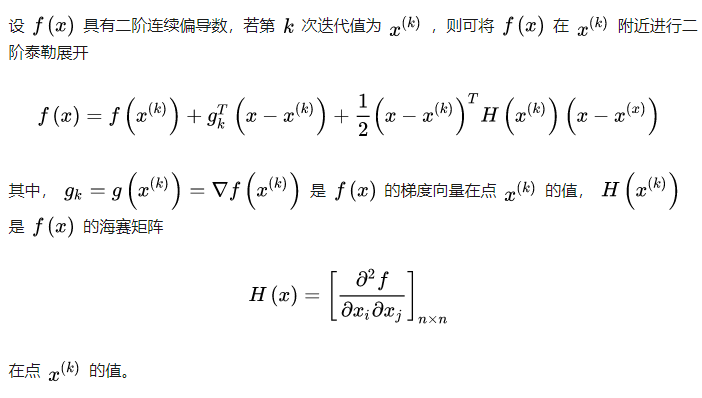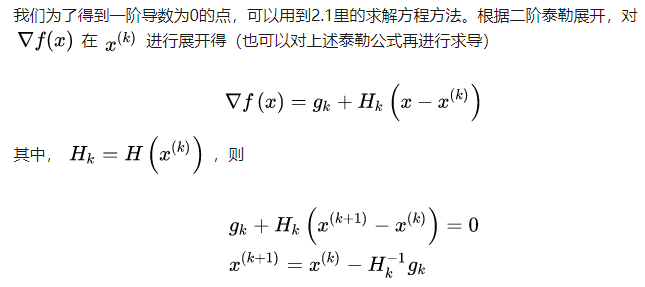(3) 梯度下降和牛顿法的区别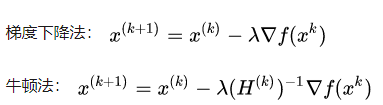梯度下降法只使用了一阶信息（其实就是泰勒一阶展开，结合更新参数向量与梯度互为反方向时，更新最快，导出的公式）；
牛顿法使用了二阶海森矩阵的逆。
因此，牛顿法迭代次数更少就能收敛了。绿色为牛顿法，红色为梯度下降法。

### 4. 拟牛顿法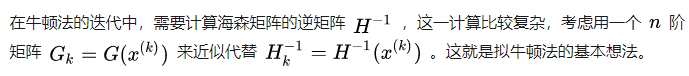也就是在迭代中，正向进行迭代而不用求逆。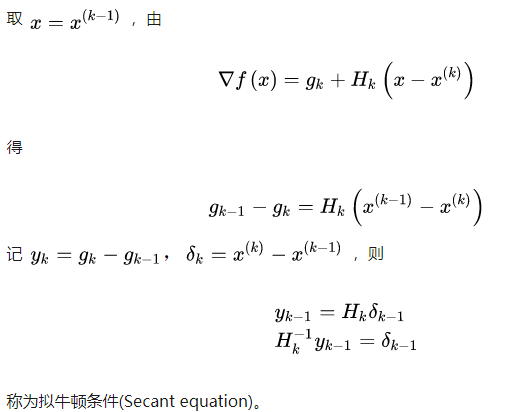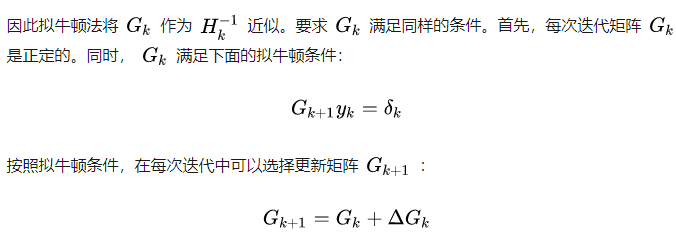#### 4.1 L-BFGS算法

一种非常流行和成功的拟牛顿法
Limited memory Broyden–Fletcher–Goldfarb–Shanno (BFGS) algorithm简单的思路是，之前的拟牛顿法需要保持矩阵，这里只需要使用向量 s, q 即可，节约内存开销。

### 5. 共轭梯度法

共轭梯度法是介于梯度下降法与牛顿法之间的一个方法，是一个一阶方法。它克服了梯度下降法收敛慢的缺点，又避免了存储和计算牛顿法所需要的二阶导数信息。

适用
二次规划问题。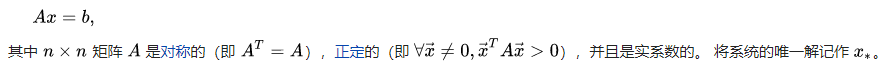思想:
共轭梯度法的基本思想是把共轭性与最速下降法相结合，利用已知点处的梯度构造一组共轭方向，并沿这组方向进行搜索，求出目标函数的极小点。根据共轭方向的基本性质，这种方法具有二次终止性。

共轭梯度算法

算法用Gram-Schmidt找 n 个共轭向量。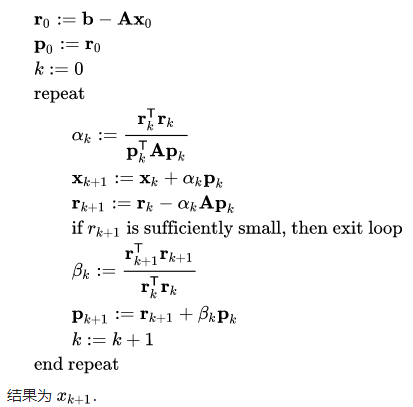### 6. 总结

在机器学习中的无约束优化算法，除了梯度下降以外，还有前面提到的最小二乘法，此外还有牛顿法和拟牛顿法。

梯度下降法和最小二乘法相比，梯度下降法需要选择步长，而最小二乘法不需要。梯度下降法是迭代求解，最小二乘法是计算解析解。如果样本量不算很大，且存在解析解，最小二乘法比起梯度下降法要有优势，计算速度很快。但是如果样本量很大，用最小二乘法由于需要求一个超级大的逆矩阵，这时就很难或者很慢才能求解解析解了，使用迭代的梯度下降法比较有优势。

梯度下降法和牛顿法/拟牛顿法相比，两者都是迭代求解，不过梯度下降法是梯度求解，而牛顿法/拟牛顿法是用二阶的海森矩阵的逆矩阵或伪逆矩阵求解。相对而言，使用牛顿法/拟牛顿法收敛更快。但是每次迭代的时间比梯度下降法长。

（1）牛顿法在什么时候只需要迭代一次就能求解，什么时候牛顿法不能适用？
对于正定二次函数，一步即可得最优解。

初始点远离最优解时，Gk不一定是正定的，则牛顿方向不一定为下降方向，其收敛性不能保证。这说明恒取步长因子为1是不合适的，应该采用一维搜索（仅当步长因子 α k {αk} 收敛1时，牛顿法才是二阶收敛的）带步长因子的牛顿法是总体收敛的。
（2）拟牛顿法解决了牛顿法哪个问题？
Hesse矩阵的计算工作量大，有时目标函数的Hesse阵很难计算。
拟牛顿法利用目标函数和一阶导数，来构造目标函数的曲率近似，而不需要明显形成Hesse阵，同时
具有收敛速度快的优点。最近开通了个公众号，主要分享推荐系统，风控等算法相关的内容，感兴趣的伙伴可以关注下。公众号相关的学习资料会上传到QQ群596506387，欢迎关注。

参考：

展开全文• 提出一种基于最大互信息系数与皮尔逊相关系数的两阶段特征选择融合算法, 并利用遗传算法对其中两个超参数自动进行优化. 第一阶段, 利用最大互信息系数获取特征和标签之间的相关性来进行特征选择; 第二阶段, 使用...
• 个设计参数下以两目标函数采用遗传算法优化进行择优
• 目标遗传算法应用于电火花机床参数优化，优化过程简单，计算结果准确，收敛速度明显加快
• MATLAB遗传算法求解数据拟合和多参数优化问题代码实例 MATLAB遗传算法求解数据拟合和多参数优化问题代码实例 遗传算法单参数/多参数寻优 多参数寻优和单一参数寻优的基本思路是相同的，不过在种群初始化时，...

MATLAB遗传算法求解数据拟合和多参数优化问题代码实例

MATLAB遗传算法求解数据拟合和多参数优化问题代码实例

遗传算法单参数/多参数寻优
多参数寻优和单一参数寻优的基本思路是相同的，不过在种群初始化时，每次需要初始化m个染色体，并且在选择、交叉、变异操作时，m个染色体是分别进行的。
步骤一：种群、染色体、基因初始化。
步骤二：计算种群中每一组染色体对应的适应度函数值。
步骤三：对种群中的染色体进行选择、交叉、变异操作，产生新的种群。
步骤四：判断是否满足终止条件？如果不满足，跳转到步骤二；如果满足，输出最优参数。
步骤五：结束。

数据拟合和多参数优化问题实例：
已知一组观测数据（x, y）满足一定的函数关系y=f(x)，求函数关系f中的未知参数。
具体到该问题为：
已知一组观测数据（x, y），如图1所示。x和y大致满足如下函数关系：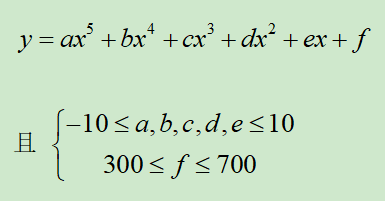（可以自己定义更复杂的关系，仅以此为例）

试求的值？ 使得x和y的拟合程度达到最佳！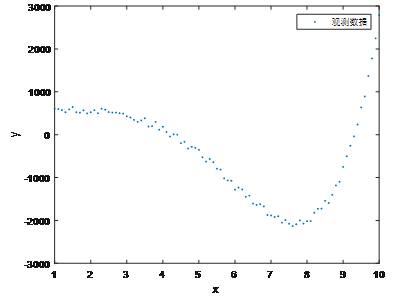图1 需要拟合的观测数据

编程求解结果：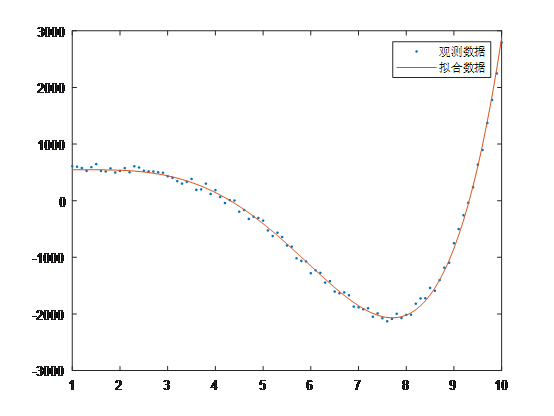待求解参数最优解分别为
a= 0.5609
b= -6.4051
c= 9.9999
d= 3.8902
e= -9.9980
f= 547.4058

展开全文matlab
• 提出一种新的自适应粒子群优化算法, 以解决梯度为基础的算法在进行多参数拟合时因各参数之间相关性较高而带来的拟合上的问题. 该粒子群优化算法采用自适应变异和动态自适应调整搜索范围、惯性权重相结合的改进策略...
• 7NSGA_2.m为目标遗传优化算法，用于整定一阶带时延的传递函数PID控制参数 Figure文件夹为各个算法整定的阶跃响应输出、最佳指标对比输出 shuju文件夹为Figure所需要的数据mat文件 ——————————————...
• 而优化又属于多目标优化过程的特点，通过人工智能技术建立了聚合物共注成型过程的多参数多目标优化数学模型，提出了基于Moldflow和多岛遗传算法的聚合物共注成型过程的多参数智能优化算法和技术。研究结果表明：经过...
• 非线性自抗扰控制器耦合参数多,常规经验整定难以获得最优参数,以至于影响控制器的控制精度.单一机制的优化算法整定出的自抗扰参数均可能是局部最优解,不能有效提高自抗扰控制器的控制精度.针对此问题, 提出一种...
• MATLAB遗传算法求解曲面拟合和多参数优化问题代码实例 MATLAB遗传算法求解曲面拟合和多参数优化问题代码实例 数据拟合和多参数优化问题实例： 已知一组观测数据（x, y, z）满足一定的函数关系z=f(x, y)，求...

MATLAB遗传算法求解曲面拟合和多参数优化问题代码实例

MATLAB遗传算法求解曲面拟合和多参数优化问题代码实例

数据拟合和多参数优化问题实例：
已知一组观测数据（x, y, z）满足一定的函数关系z=f(x, y)，求函数关系f中的未知参数。
具体到该问题为：
已知一组观测数据（x, y, z），如图1所示。x , y和z大致满足如下多项式函数关系：
（可以自己定义更复杂的关系，仅以此为例）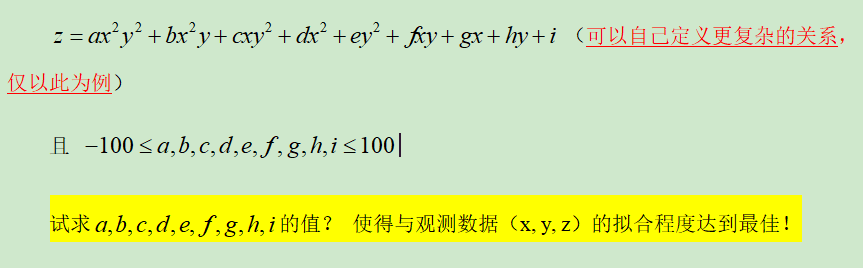试求参数的值？ 使得与观测数据（x, y, z）的拟合程度达到最佳！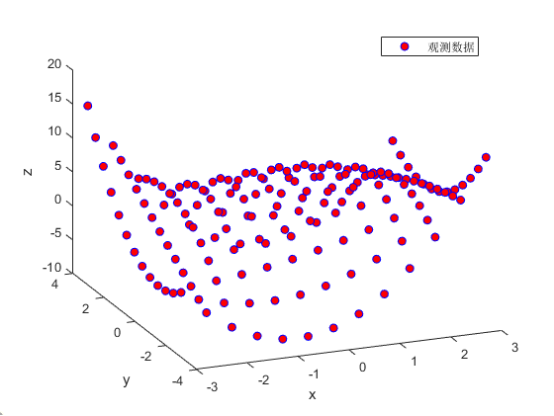图1 需要拟合的观测数据

观测数据列表（一共有13×13个数据点，每个点的x,y,z轴数据坐标如下）：
data.X 13×13的矩阵：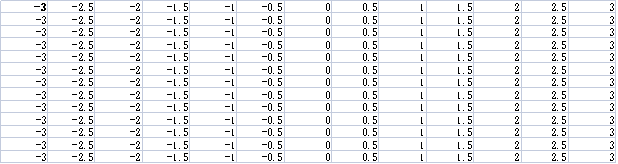data.Y 13×13的矩阵：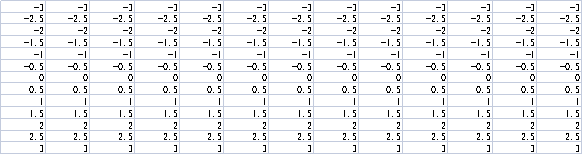data.z 13×13的矩阵：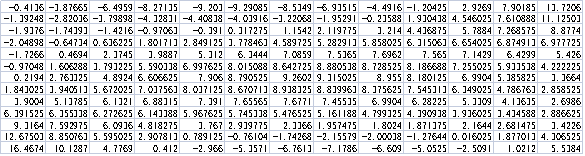编程求解结果：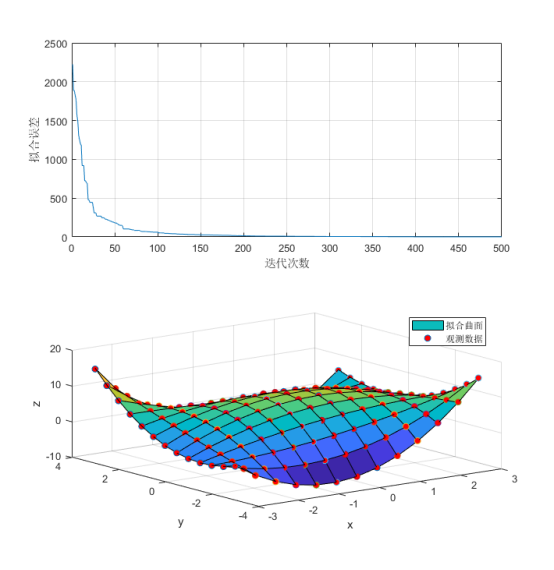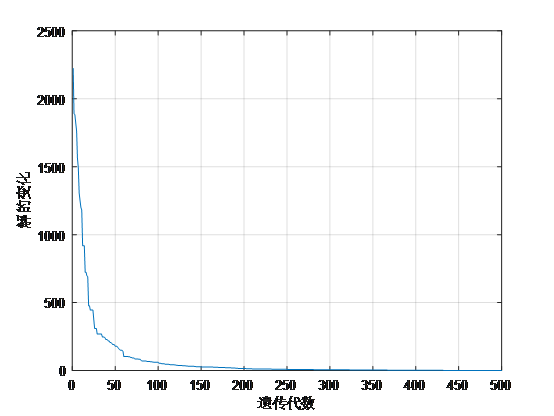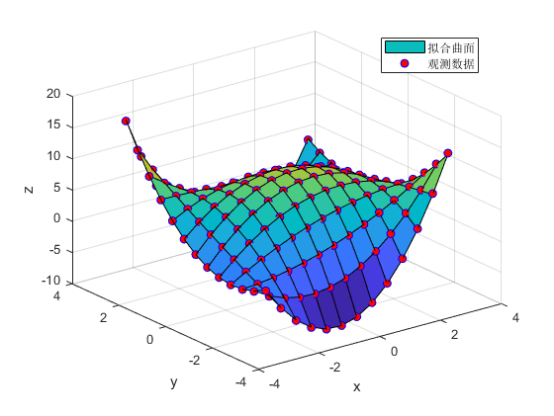待求解参数最优解分别为
a= 0.2992
b= 0.0539
c= -0.0321
d= -0.8422
e= -1.9004
f= -0.6885
g=0.5298
h=0.2797
i=9.3730

展开全文matlab python
• 基于工况优化算法的混合电动汽车参数优化.pdf
• 利用遗传算法对汽车的动力传动系进行优化，以实现汽车发动机和传动系统的最佳匹配，达到充分发挥汽车性能的目的。
• 萤火虫群优化算法（Glowworm Swarm Optimization，GSO）是目前一种新型的群智能优化方法，最初用于解决峰函数优化问题。 为了深入利用GSO算法的优势，本文分析了参数设置对GSO算法性能的影响，然后采用Likert尺度...
• 基于差分粒子群优化算法机PSS参数优化.pdf
• 通常在地球系统模式的参数优化有多个目标需要同时优化，然而目前常用的进化目标算法在地球系统模式上使用需要极高的计算代价，因此提出了一种基于多层感知机(MLP)神经网路的目标代理模式参数优化方法MO-ANN。...
• 针对在半导体制造工艺参数优化过程中缺乏直观参考的问题, 在微粒群优化算法(PSO) 和等值线理论分析 的基础上, 将PSO 与等值线矩形网格模型相结合, 提出一种全新的工艺参数窗口选择方法. 在二维标准峰函数上...
• 神经网络参数的优化方法，包括随机下降，动量优化，自适应学习率优化算法
• 为了进行超参数调优,我们一般会采用网格搜索、随机搜索、贝叶斯优化算法。在具体介绍算法之前,需要明确超参数搜索算法一般包括哪几个要素。一是目标函数,即算法需要最大化/最小化的目标二是搜索范围,一般通过上限...
• 基于遗传算法优化多尺度排列熵参数，类似于粒子群算法优化参数(Optimization of VMD parameters based on genetic algorithm) 文件列表： 基于遗传算法优化VMD参数\Code.m, 420 , 2019-12-03 基于遗传算法优化VMD...
• 以典型铁路客车的动力学模型为研究对象,以车辆最大可行速度为目标函数,采用遗传算法对其横向稳定性参数进行了最优化计算研究。...尤其是对于多参数、多峰的非线性问题,该提供了求解间题全局最优解的可能性。
• 基于云滴粒子群优化算法道次端铣削高效稳定切削参数优化方法.pdf
• 基于改进灰狼优化算法机电力系统稳定器参数优化.pdf...